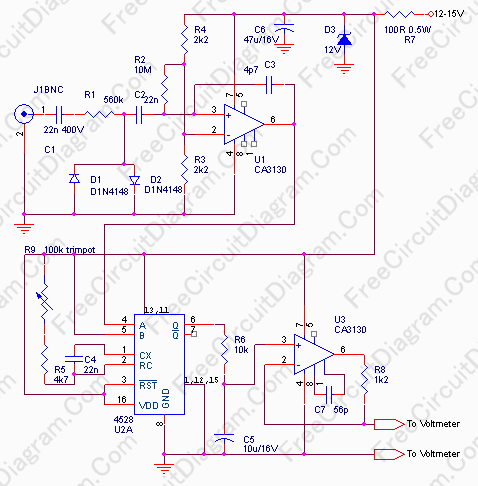## F/V Converter for Frequency Difference Measurement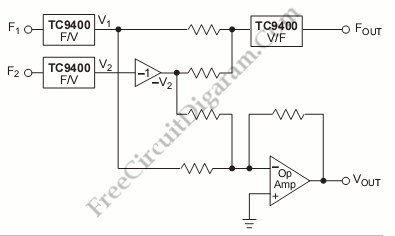This is a Frequency Difference Measurement circuit. This circuit consist of two TC9400 that is configured in the F/V mode. So, the TC9400 will convert both frequencies into proportional analog voltage(V1 and V2). This circuit also uses unity gain inverter to invert V2. After that, this circuit will add the V1 and -V2 using the summing op-amp. That operation will […]

## TC9400 Analog Frequency Meter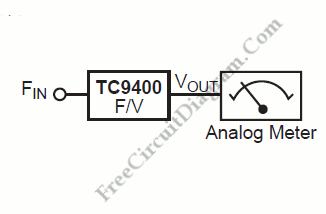Any frequency below 100kHz will be converted into an output voltage by TC9400 frequency-to-voltage converter. The TC9400 is linear to the input frequency. The equivalent frequency will be displayed on an analog meter. Here is the block diagram of the system: A frequency divider in front of the TC9400 can be used to scale the frequency down into the 100kHz […]

## Proportional FLow Rate Controller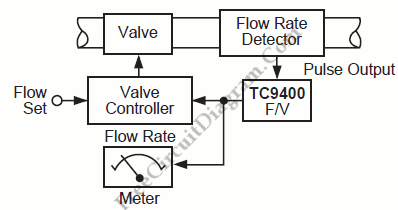Process industries deal with various control systems, and fluid mass transfer control is one of them. We can use a TC9400 F/V converter to regulate the amount of liquid or gas flowing through a pipeline. A pulse train whose frequency is proportional to the rate of flow through it is generated by the flow rate detector. This frequency is converted […]

## TC9400 Frequency-to-Voltage Converter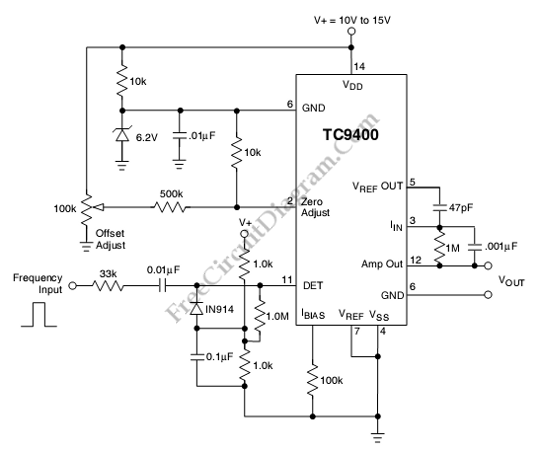This is a TC9400 Frequency-to-Voltage Converter circuit. Beside used as voltage to frequency converter, the TC9400 can also used as Frequency-to-Voltage Converter. The TC9400 will convert the input frequency waveform to output voltage linearly. This circuit has a threshold detector’s input that is used to detect each zero. The zero will cause a precise amount of charge(q=Cref Vref) to be […]

## Audio Frequency Meter Using 555 IC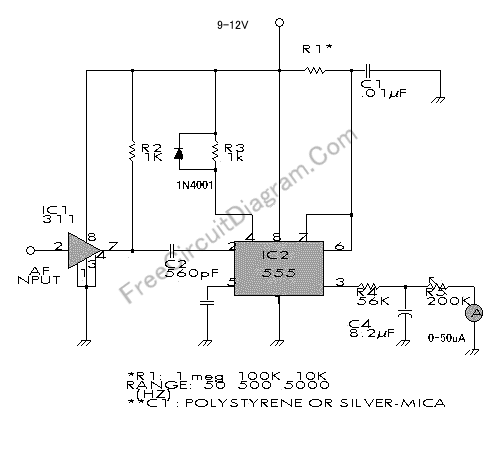This audio frequency meter uses 555 IC as a monostable multivibrator (one-shoot trigger). A monostable multivibrator can act as a frequency-to-voltage converter because it produce a fixed pulse width, with the repetition rate/density is proportional to the triggering input frequency. Here is the circuit’s schematic diagram: For resistor R1, because it set the measurement range, it’s better to use a […]

## High Precision Voltage-to-Frequency Converter (VFC) Using CA3130 Op-Amps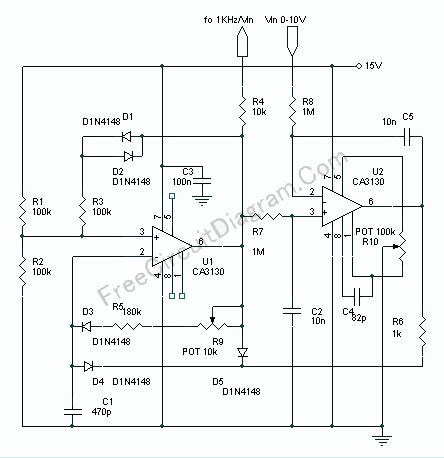Voltage to frequency is very useful in many situation, such as transmitting temperature measurement using standard voice radio transceiver. This circuit (see the circuit’s schematic diagram) uses only two CA3130 op-amps, but the performance is good enough. The linearity of the voltage-frequency transfer is better than 0.5% and the temperature coefficient is lower than 0.01% !! Assume that the output […]